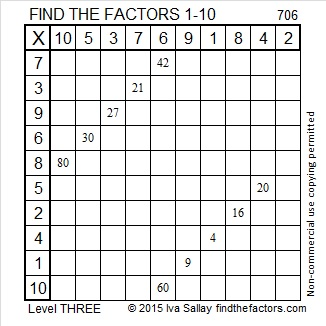# 706 and Level 3

• 706 is a composite number.
• Prime factorization: 706 = 2 x 353
• The exponents in the prime factorization are 1 and 1. Adding one to each and multiplying we get (1 + 1)(1 + 1) = 2 x 2 = 4. Therefore 706 has exactly 4 factors.
• Factors of 706: 1, 2, 353, 706
• Factor pairs: 706 = 1 x 706 or 2 x 353
• 706 has no square factors that allow its square root to be simplified. √706 ≈ 26.5706Here is today’s puzzle:Print the puzzles or type the solution on this excel file: 10 Factors 2015-12-07

———————————————————————————

Here are a few more facts about 706:

Because 353 is one of its prime factors, 706 is the hypotenuse of Pythagorean triple 450-544-706. What is the greatest common factor of those three numbers?

Not only is 706 the product of two prime palindromes (2 and 353), but 706 is also a palindrome in 2 different bases:

• 424 BASE 13; note 4(169) + 2(13) + 4(1) = 706
• 2C2 BASE 16; note 2(256) + 12(16) + 2(1) = 706

———————————————————————————

A Logical Approach to solve a FIND THE FACTORS puzzle: Find the column or row with two clues and find their common factor. (None of the factors are greater than 10.)  Write the corresponding factors in the factor column (1st column) and factor row (top row).  Because this is a level three puzzle, you have now written a factor at the top of the factor column. Continue to work from the top of the factor column to the bottom, finding factors and filling in the factor column and the factor row one cell at a time as you go.This site uses Akismet to reduce spam. Learn how your comment data is processed.